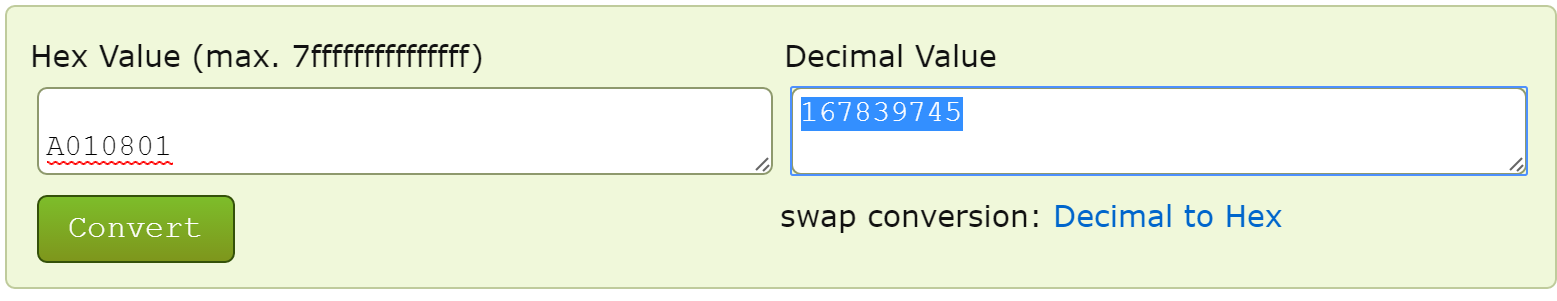This page goes over changing the alarm sounds over Garage Door Controller and form part of the larger Garage Door Controller user guide.

Configure Garage Door Controller Parameters 37-40 sounds.

It may be easier to visualize in hex rather than decimal, and if you have to input a decimal value, use a hexidecimal to decimal converter at the end.

0xYYZZVVNN

Where:

ZZ is value 2 (alarm sound)

VV is value 3 (volume)

NN is value 4 (enable/disable

So lets say i want value 1 = 10, value2 = 1, value 3 = 8, value 4 = 1 (default values for param 37)

Note - in hex, A = 10, B = 11, C = 12, D = 13, E = 14, F = 15

YY = 0A (blinking rate of 10)

ZZ = 01 (sound 1 selected)

VV = 08 (volume set to 8)

NN = 01 (enabled)

Param 37 [4 byte hex] = 0x0A010801 or 0xA010801 (most likely you will be inputting it as A010801)

If you need to input a decimal value use this calculator here: http://www.binaryhexconverter.com/hex-to-decimal-converter which should give you the decimal value from your hexidecimal value.Once you save the setting, move the sensor from close to open back to close status to save the configuration changes, then test for the changes.

-

How to completely disable the alarm sound.

If you want to know specific settings on how to disable the Garage Door Controller alarm, please refer to this link here: https://aeotec.freshdesk.com/solution/articles/6000131922-disable-alarm-sound-in-the-garage-door-controller